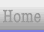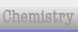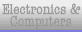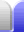# Pythagorean Triples

### More on Pythagorean Triples

A Pythagorean triple is a set of three numbers a, b and c such that:

a2 + b2 = c2

In other words, three natural numbers that satisfy Pythagoras' theorem.

Pythagorean triples consists of either all even numbers, or two odd numbers and an even number, where one of a or b will be even.

Back in 300 BC a Greek guy named Euclid came up with a way of constructing a set of Pythagorean triples. Take m and n, which can be any two whole numbers. Then a, b, and c of a Pythagorean triple are:

a = n2 - m2

b = 2nm

c = n2 + m2

(Yes, a is the difference of two squares, while c is the sum of those two squares.)

There are 16 Pythagorean triples where all the digits are less than 100. Note the pattern where the first of the triple is odd.

 c = b + 1 c = b + 2 (3, 4, 5) (5, 12, 13) (7, 24, 25) (8, 15, 17) (9, 40, 41) (11, 60, 61) (12, 35, 37) (13, 84, 85) (16, 63, 65) Others: (20, 21, 29) (28, 45, 53) (33, 56, 65) (36, 77, 85) (39, 80, 89) (48, 55, 73) (65, 72, 97)

Here are all the Pythagorean triples where a, b, and c are less than 1,000 (multiples are not included), with the same c=b+1 pattern moved to the front of the rows.

(3,4,5)
(5,12,13)
(7,24,25) (8,15,17)
(9,40,41)
(11,60,61) (12,35,37)
(13,84,85)
(15,112,113) (16,63,65)
(17,144,145)
(19,180,181) (20,21,29) (20,99,101)
(21,220,221)
(23,264,265) (24,143,145)
(25,312,313)
(27,364,365) (28,45,53) (28,195,197)
(29,420,421)
(31,480,481) (32,255,257) (33,56,65)
(33,544,545)
(35,612,613) (36,77,85) (36,323,325)
(37,684,685) (39,80,89)
(39,760,761) (40,399,401)
(41,840,841)
(43,924,925) [This is the last in the format c=b+1 with b and c less than 1,000.]

(44,117,125) (44,483,485) (48,55,73) (48,575,577) (51,140,149) (52,165,173) (52,675,677) (56,783,785) (57,176,185) (60,91,109) (60,221,229) (60,899,901) (65,72,97) (68,285,293) (69,260,269) (75,308,317) (76,357,365) (84,187,205) (84,437,445) (85,132,157) (87,416,425) (88,105,137) (92,525,533) (93,476,485) (95,168,193) (96,247,265) (100,621,629) (104,153,185) (105,208,233) (105,608,617) (108,725,733) (111,680,689) (115,252,277) (116,837,845) (119,120,169) (120,209,241) (120,391,409) (123,836,845) (124,957,965) (129,920,929) (132,475,493) (133,156,205) (135,352,377) (136,273,305) (140,171,221) (145,408,433) (152,345,377) (155,468,493) (156,667,685) (160,231,281) (161,240,289) (165,532,557) (168,425,457) (168,775,793) (175,288,337) (180,299,349) (184,513,545) (185,672,697) (189,340,389) (195,748,773) (200,609,641) (203,396,445) (204,253,325) (205,828,853) (207,224,305) (215,912,937) (216,713,745) (217,456,505) (220,459,509) (225,272,353) (228,325,397) (231,520,569) (232,825,857) (240,551,601) (248,945,977) (252,275,373) (259,660,709) (260,651,701) (261,380,461) (273,736,785) (276,493,565) (279,440,521) (280,351,449) (280,759,809) (287,816,865) (297,304,425) (300,589,661) (301,900,949) (308,435,533) (315,572,653) (319,360,481) (333,644,725) (336,377,505) (336,527,625) (341,420,541) (348,805,877) (364,627,725) (368,465,593) (369,800,881) (372,925,997) (385,552,673) (387,884,965) (396,403,565) (400,561,689) (407,624,745) (420,851,949) (429,460,629) (429,700,821) (432,665,793) (451,780,901) (455,528,697) (464,777,905) (468,595,757) (473,864,985) (481,600,769) (504,703,865) (533,756,925) (540,629,829) (555,572,797) (580,741,941) (615,728,953) (616,663,905) (696,697,985)

By the way, there are also Pythagorean quadruples.

a2 + b2 + c2 = d2

32 + 82 + 362 = 372

(1,2,2,3) (2,3,6,7) (1,4,8,9) (4,4,7,9)
(2,6,9,11) (6,6,7,11) (3,4,12,13) (2,5,14,15) (2, 10, 11, 15) (1,12,12,17) (8,9,12,17) (1,6,18,19) (6,6,17,19) (6,10,15,19) (4,5,20,21) (4,8,19,21) (4,13,16,21) (8,11,16,21) (3,6,22,23) (3,14,18,23) (6,13,18,23) (9, 12, 20, 25) (12, 15, 16, 25) (2,7,26,27) (2,10,25,27) (2,14,23,27) (7,14,22,27) (10,10,23,27) (3,16,24,29) (11,12,24,29) (12,16,21,29)

### Fermat's Last Theorem

One of the famous puzzles in mathematics is known as Fermat's Last Theorem. The theorem itself basically states that you can't do a3 + b3 = c3 for any whole numbers, or for any higher powers (eg, a4 + b4 = c4, etc), either.

If an integer n is greater than 2, then the equation an + bn = cn
has no solutions in non-zero integers a, b, and c.

It was long thought to be true, but how could it be proved?

In 1637 a mathematician named Pierre de Fermat left a note (in Latin) in the margin of a book saying "I have a truly marvellous proof of this proposition which this margin is too narrow to contain."

All Fermat's other theorems were (eventually) proved or disproved, but this one held on, and defied proof for hundreds of years. Fermat did prove the case for n=4, which reduced the problem to proving the theorem for prime numbers. Wikipedia:

Over the next two centuries (1637–1839), the conjecture was proven for only the primes 3, 5, and 7, although Sophie Germain proved a special case for all primes less than 100. In the mid-19th century, Ernst Kummer proved the theorem for a large (probably infinite) class of primes known as regular primes. Building on Kummer's work and using sophisticated computer studies, other mathematicians were able to prove the conjecture for all odd primes up to four million.

But the general proof for all n still hadn't been found. And so Fermat's Last Theorem remained unproved for 358 years, when English mathematician Andrew Wiles finally published a proof in 1995.

Most mathematicians doubt that Fermat really had a proof, but the problem became the most famous unsolved – and now most famous solved – problem in mathematics. It is thought to have generated more false proofs than any other theorem in mathematics.Home Astronomy Chemistry Electronics & Computers Mathematics Physics Field Trips Turn on javascript for email link